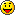How would I get my GM to do calculations for an input
Hey,

What i want:
When you type in a value (EG.1) it will, reply with the answer.

EG.1
1 = 15, 30
2 = 30, 60
3 = 45, 90
4 = 60, 120
5 = 75, 150
6 = 90, 180
7 = 105, 210
8 = 120, 240
...

Example
pawn Код:
`command(test,playerid,params[]){    new option;    if(isnull(params)) // if they didn't type anything after /test    {        return SendClientMessage(playerid, -1, "USAGE: /test <number>");    }    if(!strcmp(option, "1", true))    {        printf("15,30");        return 1;    }    if(!strcmp(option, "8", true))    {        printf("120,240");        return 1;    }    return 1;}`
But I don't want limitsso if you typed in

999 it would show
14985,29970

and if i typed in 999999
it would show
14999985,29999970
Then you need an algorithm to produce results.

pawn Код:
`new result1 = algorithm1(strval(params));new result2 = algorithm2(strval(params));stock algorithm1(value) {    value *= 20;    //More work here    return value;}stock algorithm2(value) {    value *= 25;    //More work here    return value;}`

Forum Jump:

Users browsing this thread: 1 Guest(s)